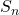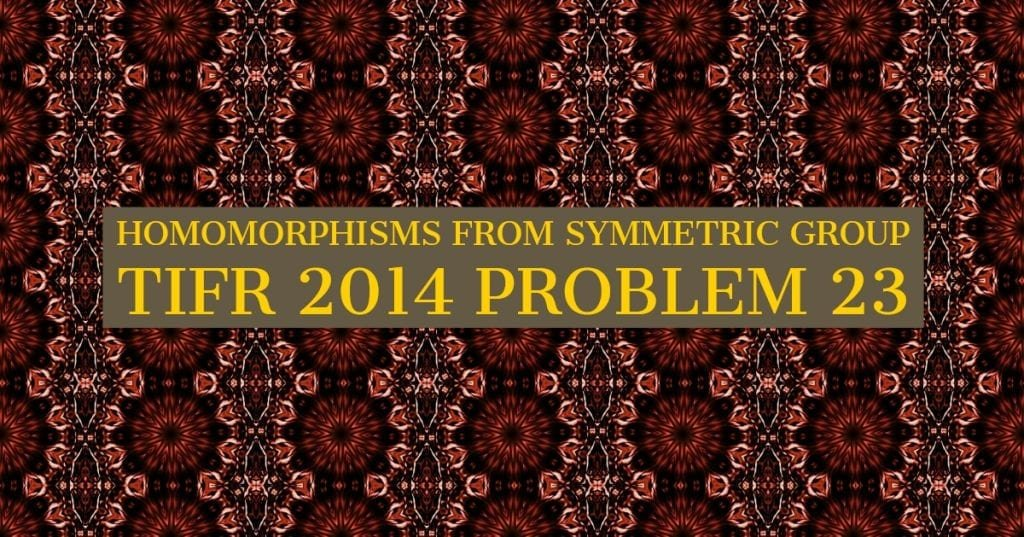How Cheenta works to ensure student success?
Explore the Back-Story

# TIFR 2014 Problem 23 Solution - Homomorphisms fromTIFR 2014 Problem 23 Solution is a part of TIFR entrance preparation series. The Tata Institute of Fundamental Research is India's premier institution for advanced research in Mathematics. The Institute runs a graduate programme leading to the award of Ph.D., Integrated M.Sc.-Ph.D. as well as M.Sc. degree in certain subjects.
The image is a front cover of a book named Contemporary Abstract Algebra by Joseph A. Gallian. This book is very useful for the preparation of TIFR Entrance.

Also Visit: College Mathematics Program

## Problem:True/False?

There exists an onto group homomorphism

A. from (S_5) to (S_4)

B. from (S_4) to (S_2)

C. from (S_5) to (\mathbb{Z}_5)

D. from (S_4) to (\mathbb{Z}_4)

## Discussion:

(S_2) is the permutation group on 2 letters. It has order 2, so it is isomorphic to (\mathbb{Z}_2).

And we know a group homomorphism from (S_n) to  (\mathbb{Z}_2), namely the signature map.

(\sigma \to 0 ) if ( \sigma ) is even

(\sigma \to 1 ) if ( \sigma ) is odd.

This map is onto. And it is a homomorphism. This is fairly well known fact so let us not prove it here.

So we know for sure that B is true.

How do we know that C is not true?

For that, suppose (\phi:S_5 \to \mathbb{Z}_5 ) be an onto group homomorphism. Apply first isomorphism theorem for groups, then (S_5/ker{\phi} ) is isomorphic to (\mathbb{Z}_5). That implies their orders are same. And (|G/H|=|G|/|H| ) so we get (|ker{\phi}| = 4! =24 ).

Now remember that (ker{\phi} ) is a normal subgroup of (S_5). Also, notice that any 4-cycle in (S_5) must go to the identity of (\mathbb{Z}_5) since the order of image must be divisible by the order of domain which in the case of 4-cycles are 4. So the image of any 4-cycle must have order 1,2 or 4 in (\mathbb{Z}_5). But out of these, only order 1 is possible in (\mathbb{Z}_5). Hence the image of any 4-cycle is the identity. So, the kernel must contain all the 4-cycles in (S_5). Now we count the number of 5-cycles in (S_5).

There are ({{5}\choose{4}} ) ways to choose 4 elements out of 1,2,3,4,5. Next, given any 4 elements, we can always write them in increasing order. Let's say we chose 2,4,5,3. Then we write this as 2,3,4,5. Now to count the number of possible 4-cycles (distinct) we fix 2 in the first position and permute the rest. Each of these permutations will give a different 4-cycle. There are (3!) such permutations. So in total, there are ({{5}\choose{4}}\times 3! =30 ) 4-cycles in (S_5). This is more than our cardinality of (ker{\phi}). This is a contradiction.

So option C is false.

The same kind of argument will apply to option D as well. Here one can consider the 3 cycles in (S_4) and get a contradiction as above.

For option A, consider the 5-cycles in (S_5). They must map to identity (because of order reasons as above). And there are (4!=24) 5-cycles (start the cycles with 1, permute rest). But first isomorphism theorem will give the cardinality of the kernel as (\frac{5!}{4!}=5), a contradiction. This disproves A.

## Helpdesk

• What is this topic:Abstract Algebra
• What are some of the associated concept: Permutation Group, Homeomorphism
• Book Suggestions: Contemporary Abstract Algebra by Joseph A. GallianTIFR 2014 Problem 23 Solution is a part of TIFR entrance preparation series. The Tata Institute of Fundamental Research is India's premier institution for advanced research in Mathematics. The Institute runs a graduate programme leading to the award of Ph.D., Integrated M.Sc.-Ph.D. as well as M.Sc. degree in certain subjects.
The image is a front cover of a book named Contemporary Abstract Algebra by Joseph A. Gallian. This book is very useful for the preparation of TIFR Entrance.

Also Visit: College Mathematics Program

## Problem:True/False?

There exists an onto group homomorphism

A. from (S_5) to (S_4)

B. from (S_4) to (S_2)

C. from (S_5) to (\mathbb{Z}_5)

D. from (S_4) to (\mathbb{Z}_4)

## Discussion:

(S_2) is the permutation group on 2 letters. It has order 2, so it is isomorphic to (\mathbb{Z}_2).

And we know a group homomorphism from (S_n) to  (\mathbb{Z}_2), namely the signature map.

(\sigma \to 0 ) if ( \sigma ) is even

(\sigma \to 1 ) if ( \sigma ) is odd.

This map is onto. And it is a homomorphism. This is fairly well known fact so let us not prove it here.

So we know for sure that B is true.

How do we know that C is not true?

For that, suppose (\phi:S_5 \to \mathbb{Z}_5 ) be an onto group homomorphism. Apply first isomorphism theorem for groups, then (S_5/ker{\phi} ) is isomorphic to (\mathbb{Z}_5). That implies their orders are same. And (|G/H|=|G|/|H| ) so we get (|ker{\phi}| = 4! =24 ).

Now remember that (ker{\phi} ) is a normal subgroup of (S_5). Also, notice that any 4-cycle in (S_5) must go to the identity of (\mathbb{Z}_5) since the order of image must be divisible by the order of domain which in the case of 4-cycles are 4. So the image of any 4-cycle must have order 1,2 or 4 in (\mathbb{Z}_5). But out of these, only order 1 is possible in (\mathbb{Z}_5). Hence the image of any 4-cycle is the identity. So, the kernel must contain all the 4-cycles in (S_5). Now we count the number of 5-cycles in (S_5).

There are ({{5}\choose{4}} ) ways to choose 4 elements out of 1,2,3,4,5. Next, given any 4 elements, we can always write them in increasing order. Let's say we chose 2,4,5,3. Then we write this as 2,3,4,5. Now to count the number of possible 4-cycles (distinct) we fix 2 in the first position and permute the rest. Each of these permutations will give a different 4-cycle. There are (3!) such permutations. So in total, there are ({{5}\choose{4}}\times 3! =30 ) 4-cycles in (S_5). This is more than our cardinality of (ker{\phi}). This is a contradiction.

So option C is false.

The same kind of argument will apply to option D as well. Here one can consider the 3 cycles in (S_4) and get a contradiction as above.

For option A, consider the 5-cycles in (S_5). They must map to identity (because of order reasons as above). And there are (4!=24) 5-cycles (start the cycles with 1, permute rest). But first isomorphism theorem will give the cardinality of the kernel as (\frac{5!}{4!}=5), a contradiction. This disproves A.

## Helpdesk

• What is this topic:Abstract Algebra
• What are some of the associated concept: Permutation Group, Homeomorphism
• Book Suggestions: Contemporary Abstract Algebra by Joseph A. Gallian

This site uses Akismet to reduce spam. Learn how your comment data is processed.

### Knowledge Partner